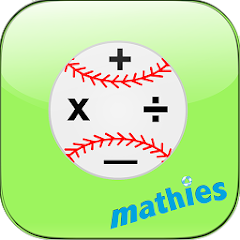# Catch Ball Ops by mathies

1K+Everyone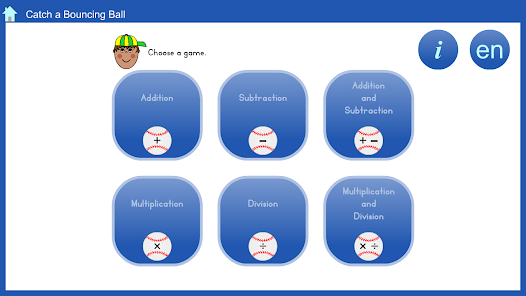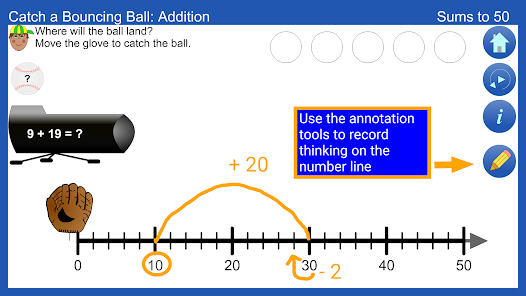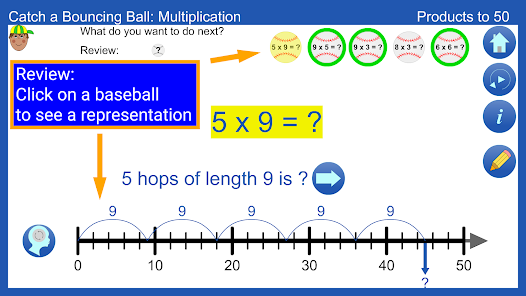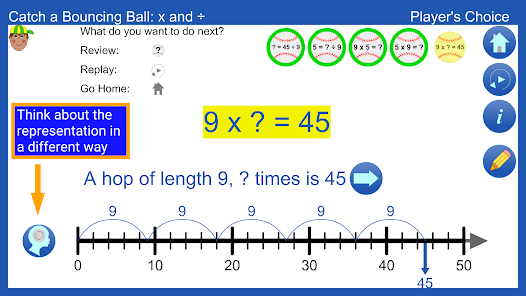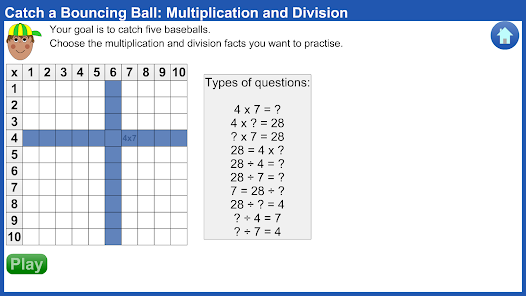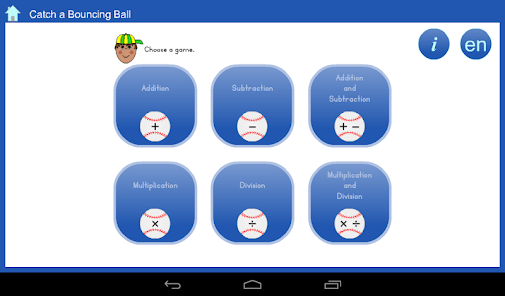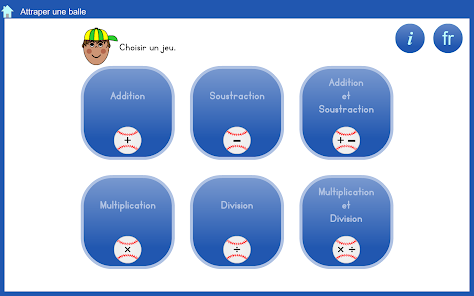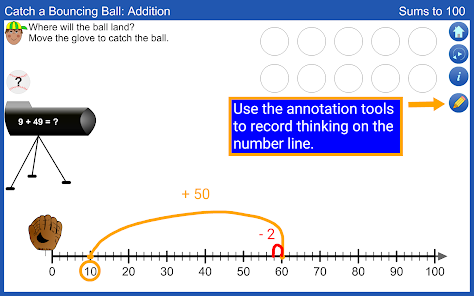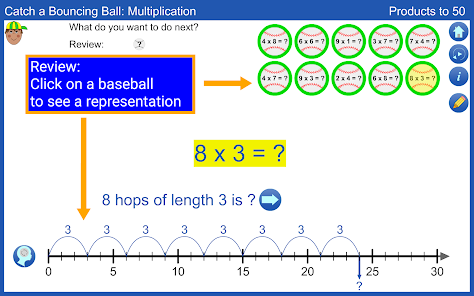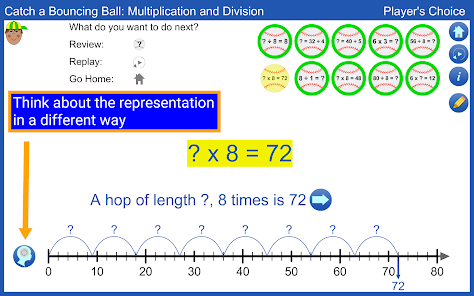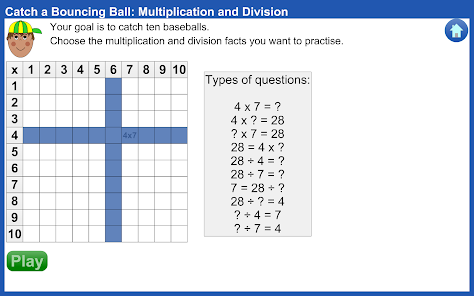Catch a Bouncing Ball Operations by mathies is a game that provides opportunities to practise the fundamental skills of addition, subtraction, multiplication or division. For single operation games the unknown value is the result (e.g., 3 + 5 = ?). For mixed operation games the position of the unknown value changes (e.g., 3 + ? = 8). The player determines the unknown value and drags the glove to the correct answer on the number line.

Mathematical Content:
• Solve problems involving addition, subtraction, multiplication and division of whole numbers using a variety of mental strategies
• Determine the missing number in an equation involving addition, subtraction, multiplication or division
• Use the identity, commutative and associative properties to facilitate computation

Features:
• Choose the operation and size of numbers to practise.
• Use the built-in annotation tool and the number line to record your thinking.
• Use the change thinking button to see alternate methods at the end of a game.
Updated on
Jun 24, 2019

## Data safety